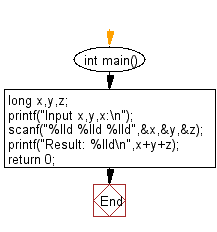﻿ C Program: Calculate for each pair of integers x, y and z - w3resource

# C Exercises: Calculate for each pair of integers x, y and z

## C Basic Declarations and Expressions: Exercise-89 with Solution

Write a C program to calculate (x + y + z) for each pair of integers x, y and z where -2^31 <= x, y, z<= 2^31-1 .

Sample Solution:

C Code:

``````#include <stdio.h>

int main()
{
// Declare variables
long long x, y, z;

// Prompt the user for input
printf("Input x, y, z:\n");

scanf("%lld %lld %lld", &x, &y, &z);

// Calculate and print the result
printf("Result: %lld\n", x + y + z);

return 0; // Indicate successful execution of the program
}
``````

Sample Output:

```Input x,y,x:
Result: 140733606875472
```

Flowchart:C programming Code Editor:

What is the difficulty level of this exercise?

Test your Programming skills with w3resource's quiz.

﻿